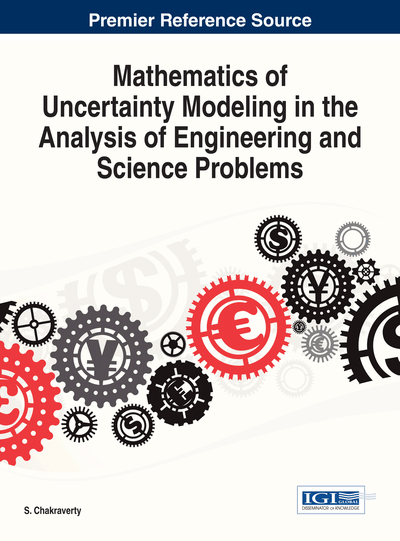# MV-Partitions and MV-Powers

C. Drossos (University of Patras, Greece) and P. L. Theodoropoulos (Educational Counselor, Greece)
DOI: 10.4018/978-1-4666-4991-0.ch011
Available
\$29.50
No Current Special Offers

## Abstract

In this chapter, the authors generalize the Boolean partition to semisimple MV-algebras. MV-partitions together with a notion of refinement is tantamount a construction of an MV-power, analogous to Boolean power construction (Mansfield, 1971). Using this new notion we introduce the corresponding theory of MV-powers.
Chapter Preview
Top

## 1. Introduction

In this introduction we should like to touch on some points that will help the readers to comprehend the contents of the Chapter.

### 1.1 Interpretations and the Change of Point of View

In modern mathematics we usually use Set Theory to express mathematical notions. In Set theory, as such there, is no uncertainty involved. However we usually have the habit to interpret some of the set-theoretic notions, in such a way that we model uncertainty. For example the functionis a well-defined entity in Set Theory, and no uncertainty is connected with such a function. However we may interpret this as a generalized indicator or a membership function of a fuzzy set. This change of point of view leads to Fuzzy Set theory. Similarly a real number, is interpreted in fuzzy set theory as a fuzzy real number. Related to the change of point of view the following Dieudonne’s saying is instructive: “What changes in Mathematics, as in all other Sciences, is the point of view from which results already acquired, are assessed. ”

The change of point of view essentially is connected with interpretations from one model to another. Think, for example, the interpretation of Euclidean terms in Riemannian geometry. To give a technical development of the intuition of interpretations it is a hard work, see e.g. (W. Hodges, 1993, Ch. 5).

Similarly, a functor, e.g. going from the category of Topological Spaces to the Category of Groups, can be construe as a change of point of view, from a topological-geometrical to algebraic.

### 1.2 Generalized Elements and Generalized Properties

Since functions play a very important role in changing a point of view from a classical set theoretic model to a non-classical one, we should like to comment on the two basic interpretations for the concept of function:

In this way partitions and generalized properties are strongly interconnected.

One general approach to this and similar problems is through Category Theory. We know that there is a “reversing the arrows duality” between subsets and partitions see (Lawvere & Rosebruch, 2003 and Ellerman, 2010). In particular we are interesting in the duality of “generalized elements” and “generalized properties” (Lawvere & Rosebruch, 2003).

We would like to present this reversing the arrows duality in some characteristic examples.

In this way we may have a notion of a graph of an arrow in any category with finite limits.

To reveal the connection of Boolean powers with the reversing the arrows duality we have to calculate explicitly the reverse of the function.

On the domain A we define the equivalence relation:

Let now,the equivalence classes of the relation. Then it is clear thatconstitutes a partition of A. Thus we have the following function:

Let,

We can repeat the above construction in the categories of probability spaces and in the category of probability algebras as well, giving the essence of the construction of Boolean powers.

## Complete Chapter List

Search this Book:
Reset